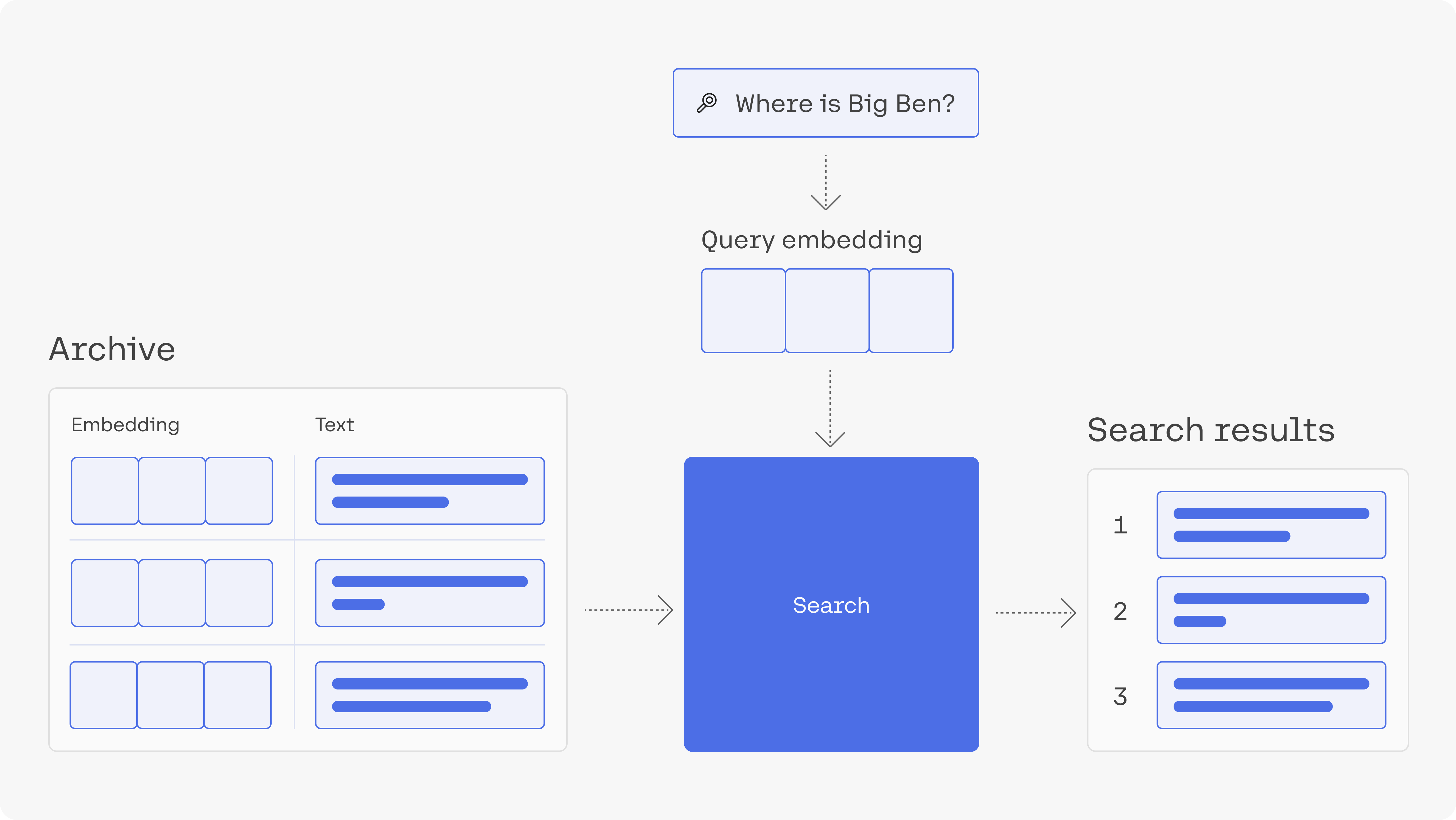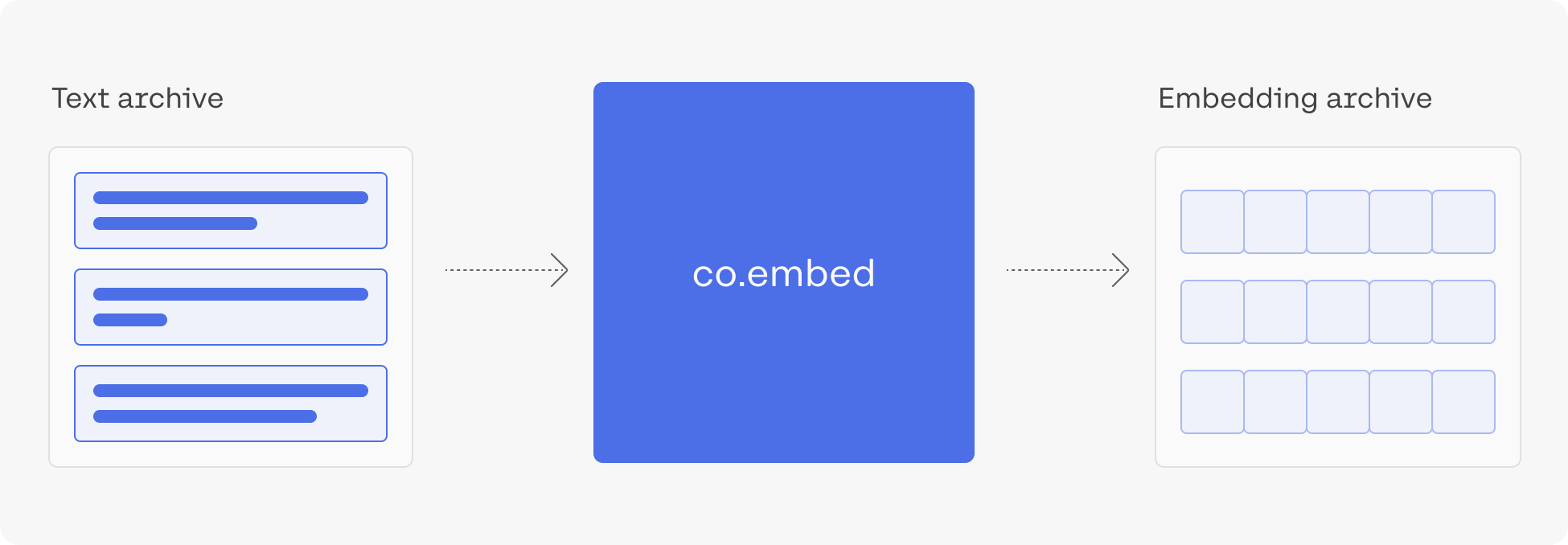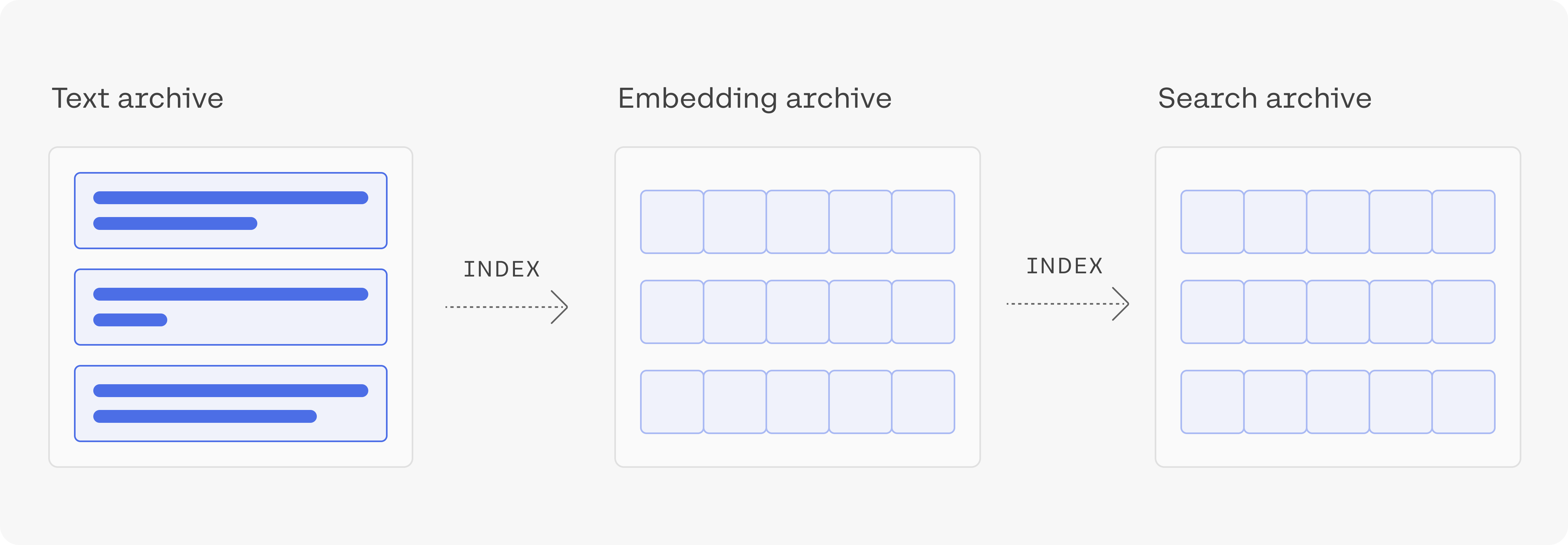# Semantic Search

### 📘This Guide Uses the Embed Endpoint.

Language models give computers the ability to search by meaning and go beyond searching by matching keywords. This capability is called semantic search.In this article, we'll build a simple semantic search engine. The applications of semantic search go beyond building a web search engine. They can empower a private search engine for internal documents or records. It can be used to power features like StackOverflow's "similar questions" feature.

You can find the code in the notebook and colab.

### Contents

Get the archive of questions
Embed the archive
Search using an index and nearest neighbour search
Visualize the archive based on the embeddings.

### 📘New to Cohere?

Representation models with billions of parameters.

``````# Install Cohere for embeddings, Umap to reduce embeddings to 2 dimensions,
# Altair for visualization, Annoy for approximate nearest neighbor search
!pip install cohere umap-learn altair annoy datasets tqdm
``````

#### 1a. Import the Necessary Dependencies to Run this Example

``````#title Import libraries (Run this cell to execute required code) {display-mode: "form"}

import cohere
import numpy as np
import re
import pandas as pd
from tqdm import tqdm
import umap
import altair as alt
from sklearn.metrics.pairwise import cosine_similarity
from annoy import AnnoyIndex
import warnings
warnings.filterwarnings('ignore')
pd.set_option('display.max_colwidth', None)
``````

### 2. Get the Archive of Questions

We'll use the trec dataset which is made up of questions and their categories.

``````# Get dataset
# Import into a pandas dataframe, take only the first 1000 rows
df = pd.DataFrame(dataset)[:1000]
# Preview the data to ensure it has loaded correctly
``````
label-coarselabel-finetext
000How did serfdom develop in and then leave Russia ?
111What films featured the character Popeye Doyle ?
222How can I find a list of celebrities ' real names ?
333What fowl grabs the spotlight after the Chinese Year of the Monkey ?
444What is the full form of .com ?
555What contemptible scoundrel stole the cork from my lunch ?
666What team did baseball 's St. Louis Browns become ?
777What is the oldest profession ?
888What are liver enzymes ?
999Name the scar-faced bounty hunter of The Old West .

### 3. Embed the ArchiveLet's now embed the text of the questions.

To get a thousand embeddings of this length should take a few seconds.

``````# Paste your API key here. Remember to not share publicly
api_key = ''

# Create and retrieve a Cohere API key from dashboard.cohere.ai/welcome/register
co = cohere.Client(api_key)

# Get the embeddings
embeds = co.embed(texts=list(df['text']),
model='embed-english-v2.0').embeddings
``````

### 4. Build the Index, search Using an Index and Conduct Nearest Neighbour SearchLet's build an index using the library called annoy. Annoy is an library created by Spotify to do nearest neighbour search; nearest neighbour search is an optimization problem of finding the point in a given set that is closest (or most similar) to a given point.

``````# Create the search index, pass the size of embedding
search_index = AnnoyIndex(np.array(embeds).shape, 'angular')
# Add all the vectors to the search index
for i in range(len(embeds)):
search_index.build(10) # 10 trees
search_index.save('test.ann')
``````

After building the index, we can use it to retrieve the nearest neighbours either of existing questions (section 3.1), or of new questions that we embed (section 3.2).

#### 4a. Find the Neighbours of an Example from the Dataset

If we're only interested in measuring the similarities between the questions in the dataset (no outside queries), a simple way is to calculate the similarities between every pair of embeddings we have.

``````# Choose an example (we'll retrieve others similar to it)
example_id = 92
# Retrieve nearest neighbors
similar_item_ids = search_index.get_nns_by_item(example_id,10,
include_distances=True)
# Format and print the text and distances
results = pd.DataFrame(data={'texts': df.iloc[similar_item_ids]['text'],
'distance': similar_item_ids}).drop(example_id)
print(f"Question:'{df.iloc[example_id]['text']}'\nNearest neighbors:")
results
``````
``````# Output:
Question:'What are bear and bull markets ?'
Nearest neighbors:
``````
textsdistance
614What animals do you find in the stock market ?0.896121
137What are equity securities ?0.970260
601What is `` the bear of beers '' ?0.978348
307What does NASDAQ stand for ?0.997819
683What is the rarest coin ?1.027727
112What are the world 's four oceans ?1.049661
864When did the Dow first reach ?1.050362
547Where can stocks be traded on-line ?1.053685
871What are the Benelux countries ?1.054899

#### 4b. Find the Neighbours of a User Query

We're not limited to searching using existing items. If we get a query, we can embed it and find its nearest neighbours from the dataset.

``````query = "What is the tallest mountain in the world?"

# Get the query's embedding
query_embed = co.embed(texts=[query],
model="embed-english-v2.0").embeddings

# Retrieve the nearest neighbors
similar_item_ids = search_index.get_nns_by_vector(query_embed,10,
include_distances=True)
# Format the results
results = pd.DataFrame(data={'texts': df.iloc[similar_item_ids]['text'],
'distance': similar_item_ids})

print(f"Query:'{query}'\nNearest neighbors:")
results
``````
textsdistance
236What is the name of the tallest mountain in the world ?0.431913
670What is the highest mountain in the world ?0.436290
907What mountain range is traversed by the highest railroad in the world ?0.715265
435What is the highest peak in Africa ?0.717943
354What ocean is the largest in the world ?0.762917
412What was the highest mountain on earth before Mount Everest was discovered ?0.767649
109Where is the highest point in Japan ?0.784319
114What is the largest snake in the world ?0.789743
656What 's the tallest building in New York City ?0.793982
901What 's the longest river in the world ?0.794352

### 5. Visualize the Archive

``````#@title Plot the archive {display-mode: "form"}

# UMAP reduces the dimensions from 1024 to 2 dimensions that we can plot
reducer = umap.UMAP(n_neighbors=20)
umap_embeds = reducer.fit_transform(embeds)
# Prepare the data to plot and interactive visualization
# using Altair
df_explore = pd.DataFrame(data={'text': df['text']})
df_explore['x'] = umap_embeds[:,0]
df_explore['y'] = umap_embeds[:,1]

# Plot
chart = alt.Chart(df_explore).mark_circle(size=60).encode(
x=#'x',
alt.X('x',
scale=alt.Scale(zero=False)
),
y=
alt.Y('y',
scale=alt.Scale(zero=False)
),
tooltip=['text']
).properties(
width=700,
height=400
)
chart.interactive()
``````